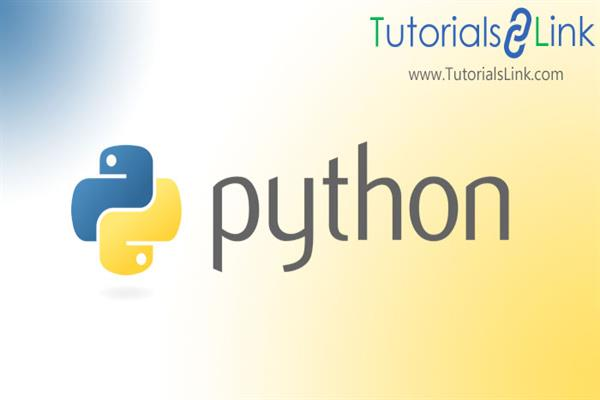Loading, please wait...# What are Python Data types?

May 17, 2020 python, Data Types, Python Data Types, 2459 Views
In this article, we will discuss the various data types used in python.

### Python Data types

Variables are containers that can hold values. These containers know as variables hold data of different types. Data Types are the classification of data items that categories the data as per the operations that can be performed or properties of the data. In technical words, data type or type is an attribute of data that tells the compiler or interpreter what the programmer is intended to do. Python automatically detects the data type of a variable by its name.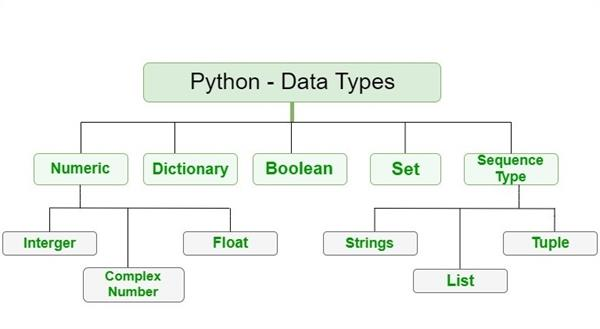### Python Data Types are categorized as:

 IMMUTABLE MUTABLE Numeric List String Dictionary Tuple Boolean

Immutable data types: These are the ones that cannot be changed after creation which means once you store this data type in some variable, its value cannot be changed.

Mutable data types: These are the data types that can be changed or altered after creation such as List, Dictionary, etc.

## Numeric Data Type:

The numeric data type is the data type that deals with numbers. It is immutable in nature. This data type can be further divided into 3 subcategories.

### Integer:

It is the data type of the whole number. In a programming language, Whole numbers are known as ‘Integer’. Any variable declared as int becomes the object of ‘int’ class. Integer can be positive or negative.

Example:

``a = 4``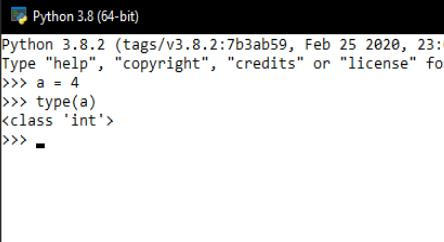The size of the integer depends on the memory available.

### Float:

The variables are the object of float class. These are the number which represents floating-point numbers. These are the real numbers and are written with a decimal point dividing the integer and fractional parts eg 9.38.

Example:

``````a = 9.38
``````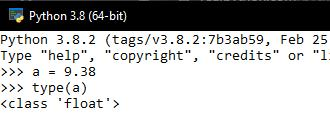Floating-point numbers the accurate up to 15 decimal places.

### Complex Number:

The variable is the object of the ‘complex’ class. It is represented in the form of ‘a+bi’ where ‘a’ is the real part and ‘b’ is the imaginary part.

Example: a= 2+3j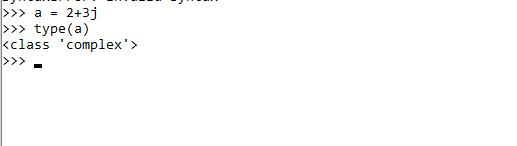## String Data Type:

The string is the name for the alphabetical data type. It stores a sequence of characters in a single object. Variable declared as the string is an object of ‘string’ class. It is represented by the ‘str’ keyword. It is enclosed between single, double, or triple quotes. It is also an immutable data type. If there is only 1 character stored, it will be represented as a string of length 1.

Example:

``a = ‘Python’``

Accessing string:

Strings can only be accessed using Indexes of the element. Indexing is the technique of assigning numbers to the alphabets so that the alphabets can be accessed later. Indexing starts from 0 and only integer numbers are allowed as an index.

 P Y t h o n 0 1 2 3 4 5

This is called positive indexing as the first alphabet is at index 0.

 P y t h o n -6 -5 -4 -3 -2 -1

This is called negative indexing.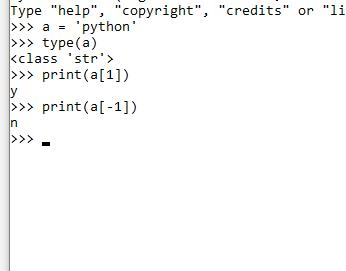In order to access the alphabets, indexes and index operator [] is used.

## Tuple Data Type:

The tuple is a collection of the ordered sequences of items the same as List. It is an immutable data type and after created cannot be modified. It cannot change dynamically and is faster than List. It is defined within parentheses where items are separated by commas. Tuple can have any number of items with different types.

Syntax:

``Variable_name = (value1,value2)``

Example

``city = (‘Delhi’,’Mumbai’)``

However, a tuple can also be created using a single item but trailing comma is mandatory. Example name =(python, )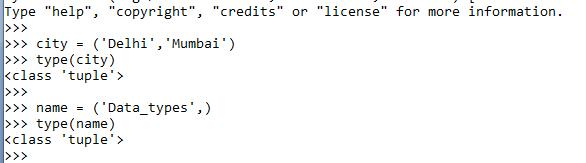Tuples are also accessed using indexing.

## Boolean Data Type:

The boolean data type is the one that has only two values as output. These are either “True” or “False”. The boolean data type has a” bool” keyword and is the object of the “bool” class. When the Boolean object is correct output is True otherwise False. To the contrary to this, non-Boolean types can also be evaluated as a bool.

‘T’ in True and ‘F’ in False must be capital in order to use as Booleans otherwise python will throw an error.

Syntax:

``variable_name = Boolean_value``

Example:

``a = True``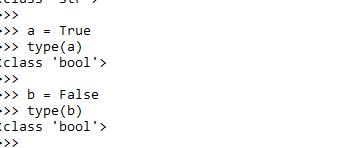## List Data Type:

The list is a mutable data type.  Just like an array (which declared in other languages), It is a collection of ordered sequences having the same or different data type, for example, a single list can have int, str, and bool values.

In a list, values are stored in order and the indexing technique is used. Index starts from ‘0’.Index operator along with the index value is used to access an item in a list.

It is defined within square brackets [], and items or values are separated by commas. As it is a mutable type, alteration in the list can be made.

Syntax:

``name _of_ the_list = [ value1,value2]``

Example:

``This_list = [‘Data’,’Types’]``

Even after the creation of the list, values can be added to the list. Built-in functions are used to add new value.

1. append(): append() is a built-in function which is used to add one element at a time, at the end of the list.
2. extend(): This function is used to add multiple values at the end of the list.
3. insert(): This function allows the addition of new value in the list at the desired location.

To remove an element from the list, built-in functions are used:

1. remove(): Remove function is used to remove the given element from the list and throw an error if the element is not found.
2. pop(): This function is used to return and remove the element from the list. By default, it removes the last element. The index is passed as an argument if the desired element is to be removed.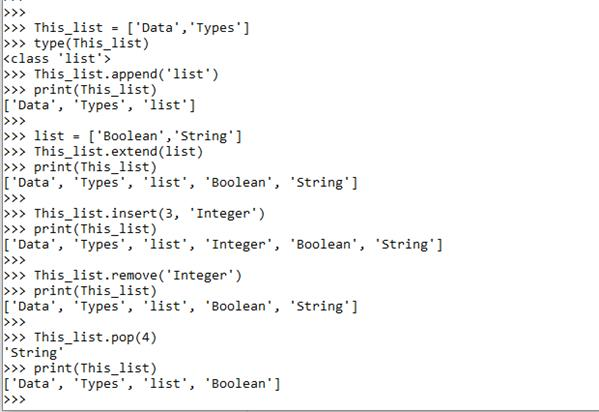## Dictionary Data Type:

Another mutable data type, It is an unordered, changeable, and Indexed collection of values. The mapping concept is used in the dictionary as it holds “key: value” pairs. This concept makes it one of the most optimized data types. Here dictionary is enclosed in brackets where every key holds one value. Key and value are separated by colon(:) and keys are separated by commas (,).  Variables are the object of ‘Dict’ class.

Syntax:

``name of the list: { key:value , key: value}``

Example:

``counting : {1: ‘one’, 2: ‘two’}``

It is case sensitive in nature so keys with the same name but the different cases will always be considered different.

Accessing an element:

get() method is used to access the pair or directly key can be passed within a square bracket.

In the dictionary, new values can be added by passing the key and value pair

Example: counting = ‘three’

So this will be added at the end of the dictionary. In order to update the existing key-value pair, an update() function is used. It will update the key-value pair if it exists otherwise creates new.

Deleting an element:

Del keyword can be used to delete a specific element or the whole dictionary. Moreover, pop() and popitem() methods are used to delete a specific element or arbitrary value from a dictionary. The clear () method is used to remove all the pairs from the dictionary.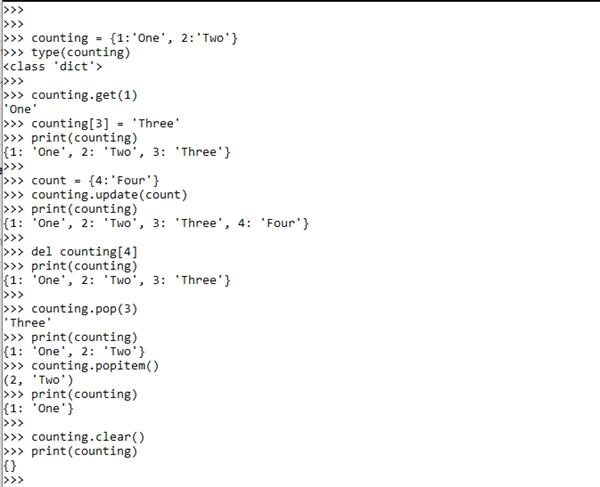These are the basic data types used in python. But you can also check the data type of a variable ant run time using built-in functions. type() function returns the type of the data and range() function returns the range of the data type used.